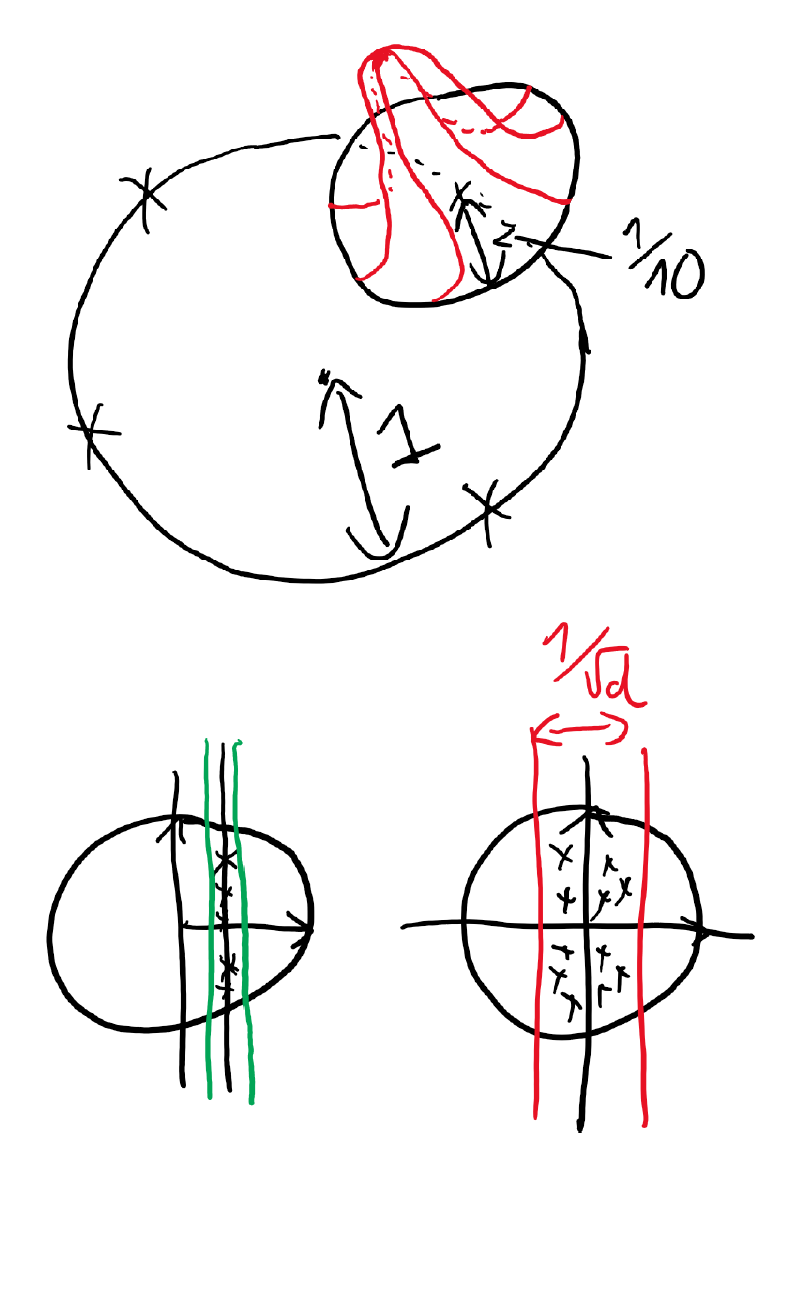# A Universal Law of Robustness via Isoperimetry## Introduction

Classically, data interpolation with a parametrized model class is possible as long as the number of parameters is larger than the number of equations to be satisfied. A puzzling phenomenon in deep learning is that models are trained with many more parameters than what this classical theory would suggest. We propose a theoretical explanation for this phenomenon. We prove that for a broad class of data distributions and model classes, overparametrization is necessary if one wants to interpolate the data smoothly. Namely we show that smooth interpolation requires d times more parameters than mere interpolation, where d is the ambient data dimension. We prove this universal law of robustness for any smoothly parametrized function class with polynomial size weights, and any covariate distribution verifying isoperimetry. In the case of two-layers neural networks and Gaussian covariates, this law was conjectured in prior work by Bubeck, Li and Nagaraj. We also give an interpretation of our result as an improved generalization bound for model classes consisting of smooth functions.